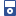Accessibility options:

# Other conic sections (ellipse, hyperbola, parabola) resources

Show me all resources applicable to

### iPOD Video (5)Conic Sections Part 1
IPOD VIDEO: In this unit we study the conic sections. These are the curves obtained when a cone is cut by a plane. We find the equations of one of these curves, the parabola, by using an alternative description in terms of points whose distances from a fixed point and a fixed line are equal. We also find the equation of a tangent to a parabola using techniques from calculus, and we use this to prove the reflective property of the parabola. This resource is released under a Creative Commons license Attribution-Non-Commercial-No Derivative Works and the copyright is held by Skillbank Solutions Ltd.Conic Sections Part 2
IPOD VIDEO: In this unit we study the conic sections. These are the curves obtained when a cone is cut by a plane. We find the equations of one of these curves, the parabola, by using an alternative description in terms of points whose distances from a fixed point and a fixed line are equal. We also find the equation of a tangent to a parabola using techniques from calculus, and we use this to prove the reflective property of the parabola. This resource is released under a Creative Commons license Attribution-Non-Commercial-No Derivative Works and the copyright is held by Skillbank Solutions Ltd.Conic Sections Part 3
IPOD VIDEO: In this unit we study the conic sections. These are the curves obtained when a cone is cut by a plane. We find the equations of one of these curves, the parabola, by using an alternative description in terms of points whose distances from a fixed point and a fixed line are equal. We also find the equation of a tangent to a parabola using techniques from calculus, and we use this to prove the reflective property of the parabola. This resource is released under a Creative Commons license Attribution-Non-Commercial-No Derivative Works and the copyright is held by Skillbank Solutions Ltd.Conic Sections Part 4
IPOD VIDEO: In this unit we study the conic sections. These are the curves obtained when a cone is cut by a plane. We find the equations of one of these curves, the parabola, by using an alternative description in terms of points whose distances from a fixed point and a fixed line are equal. We also find the equation of a tangent to a parabola using techniques from calculus, and we use this to prove the reflective property of the parabola. This resource is released under a Creative Commons license Attribution-Non-Commercial-No Derivative Works and the copyright is held by Skillbank Solutions Ltd.Conic Sections Part 5
IPOD VIDEO: In this unit we study the conic sections. These are the curves obtained when a cone is cut by a plane. We find the equations of one of these curves, the parabola, by using an alternative description in terms of points whose distances from a fixed point and a fixed line are equal. We also find the equation of a tangent to a parabola using techniques from calculus, and we use this to prove the reflective property of the parabola. This resource is released under a Creative Commons license Attribution-Non-Commercial-No Derivative Works and the copyright is held by Skillbank Solutions Ltd.

### Motivating Mathematics (1)Parabolas
This extension video mathstutor shows practical applications of parabolas such as the transmission of radio waves. This resource is released under a Creative Commons license Attribution-Non-Commercial-No Derivative Works and the copyright is held by Skillbank Solutions Ltd.

### Teach Yourself (1)Conic sections
In this unit we study the conic sections. These are the curves obtained when a cone is cut by a plane. We find the equations of one of these curves, the parabola, by using an alternative description in terms of points whose distances from a fixed point and a fixed line are equal. We also find the equation of a tangent to a parabola using techniques from calculus, and we use this to prove the reflective property of the parabola.

### Test Yourself (2)Diagnostic Test - Conic sections
This resource is released under a Creative Commons license Attribution-Non-Commercial-No Derivative Works and the copyright is held by Skillbank Solutions Ltd.Exercise - Conic sections
This resource is released under a Creative Commons license Attribution-Non-Commercial-No Derivative Works and the copyright is held by Skillbank Solutions Ltd.

### Video (1)Conic sections
In this unit we study the conic sections. These are the curves obtained when a cone is cut by a plane. We find the equations of one of these curves, the parabola, by using an alternative description in terms of points whose distances from a fixed point and a fixed line are equal. We also find the equation of a tangent to a parabola using techniques from calculus, and we use this to prove the reflective property of the parabola. (Mathtutor Video Tutorial) This resource is released under a Creative Commons license Attribution-Non-Commercial-No Derivative Works and the copyright is held by Skillbank Solutions Ltd.

Website design by Pink Mayhem, Leicester